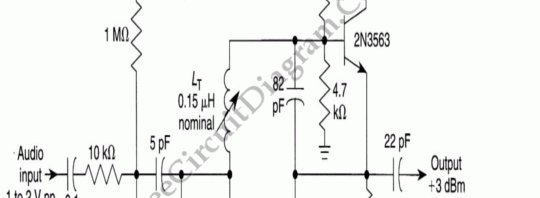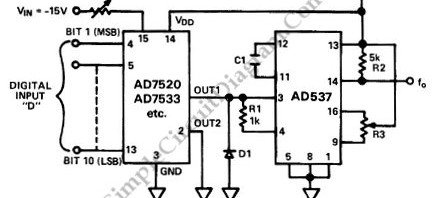## Simple Ultrasonic Wave GeneratorUltrasonic sound wave can be generated using electronic circuit. This simple electronic circuit can generate an ultrasonic wave with frequency range of 12 to 50 kHz. Its very easy and quick to make this circuit. This circuit uses NE555 IC timer to drive a speaker. SPKR1 can be piezzo tweeter, etc. If you use a moving coil tweeter, then it […]

## Frequency Modulated Oscillator (for FM Transmitter)An oscillator can be built using LC (inductor-capacitor) tank circuit. When we vary the capacity of the capacitor in LC tank circuit then the frequency of the oscillator will vary according to capacitance change.  The idea of this modulator is by employing a varactor (voltage-dependent variable capacitor) in the LC tank circuitry. As the capacitance is controlled by a voltage […]

## Voltage to Frequency Converter Using UJTThe following voltage to frequency converter (V/FC – VCO) circuit consist of a UJT (uni-junction transistor) oscillator in which the timing charge capacitor C2 is linearly depends on the input signal voltage. The voltage across resistor R5 set the charging current, which is accurately controlled by the amplifier. Here is the schematic diagram of the circuit: